Function Repository Resource:

# FourierShiftInverse

Invert a zero-frequency shift

Contributed by: Yong-an Lu
 ResourceFunction["FourierShiftInverse"][data] rearranges a zero-frequency-shifted Fourier transform data back to the original transform output. ResourceFunction["FourierShiftInverse"][data, dim] operates along the dimension dim of data.

## Details and Options

ResourceFunction["FourierShiftInverse"] is the inverse of the resource function FourierShift.
In optics, the zero-frequency term appears in the center of spectrum.
ResourceFunction["FourierShiftInverse"] typically works with InverseFourier.
InverseFourier assumes that the zero-frequency term appears at position 1 in the input list.

## Examples

### Basic Examples (5)

Swap the left and right halves of a vector:

 In:=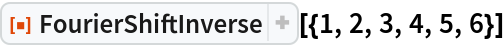Out=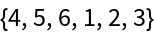If a vector has an odd number of elements, then the middle element is considered part of the right half of the vector:

 In:=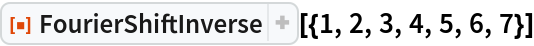Out=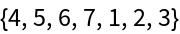Swap the first quadrant of the matrix with the third, and the second quadrant with the fourth:

 In:=Out=Swap halves of each column of matrix:

 In:=Out=Swap halves of each row of matrix:

 In:=Out=### Scope (2)

FourierShiftInverse can be applied to a multidimensional array:

 In:=Out=The dimension can be any valid part specification:

 In:=Out=In:=Out=### Applications (3)

An optical low-pass filter model:

 In:=Apply the filter to a image:

 In:=Out=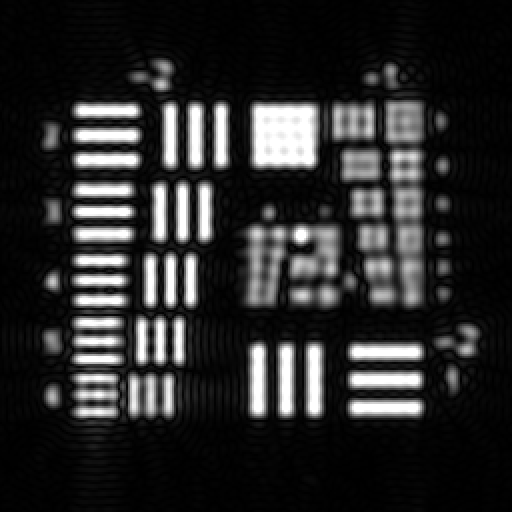### Properties and Relations (1)

FourierShiftInverse is the inverse of the resource function FourierShift:

 In:=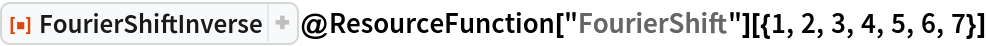Out=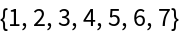### Possible Issues (1)

FourierShiftInverse does not support ragged arrays:

 In:=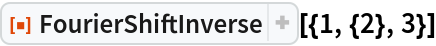Out=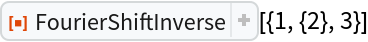Yong-an Lu

## Version History

• 1.0.0 – 11 October 2019